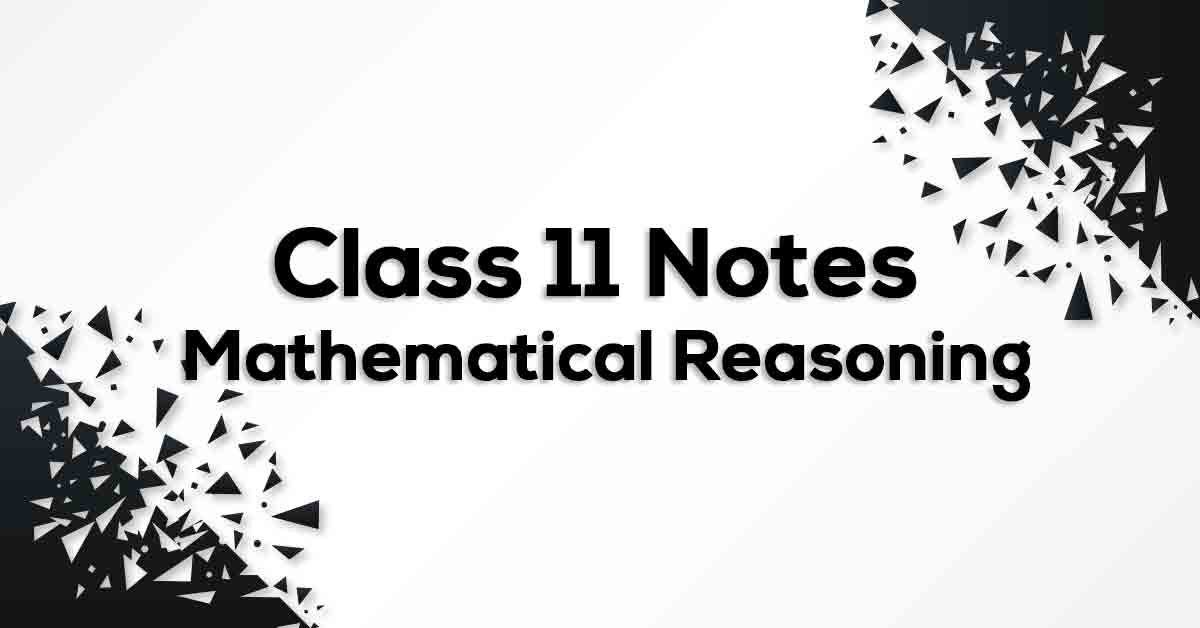Mathematical Reasoning Class 11 Notes | Vidyakul
×

# Mathematical Reasoning Class 11 Notes## Class 11 Math Chapter 14 Mathematical Reasoning Notes - PDF Download

Mathematical Reasoning Class 11 Notes is prepared strictly according to the NCERT Syllabus which not only reduces the pressure on the students but also, offer them a simple way to study or revise the chapter. These formulae are cumulated from past 15 years of examination material preferred by CBSE so that no important formulae should be left behind for the students to know and practice. Mathematical Reasoning Class 11 Notes would fuel your exam preparation and increase your self-confidence which would help you score maximum marks in the exam.

### How do you define Mathematical Reasoning?

A sentence is called a mathematically acceptable statement if it is either true or false. A Compound Statement is a statement which is made up of two or more statements. The converse of a given statement “if p, then q” is “if q, then p”. If p and q are mathematical statements, then in order to show that the statement “p and q” is true, the following steps are followed. If p and q are mathematical statements, then in order to show that the statement “p or q” is true, one must consider the following. Case 1 By assuming that p is false, show that q must be true. Case 2 By assuming that q is false, show that p must be true.